### Lazuli and the Numbers 6+

Three different degrees of difficulty each
Foundations of counting, characteristics, quantities, symmetry, memory, perception, and geometry
For ages approx. six years and over

### Angels

Instruction e.g.: "There are 5 angels!
Look carefully where they are flying!"
Basic Mathematical Skills:
Basic Non-Numerical Skills: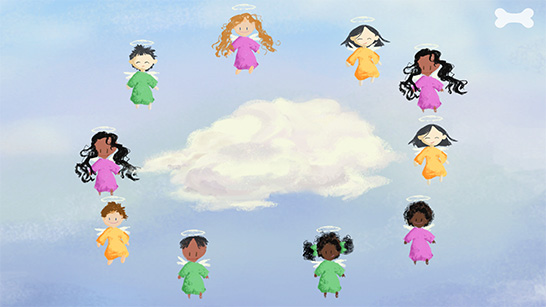### Princess

Instruction e.g.: "Tap on the frog on the left!"
Basic Non-Numerical Skills:### Colored Fields

Instruction e.g.: "Push all the black dots onto the two colored fields!
Each field should have the same number of dots."
Basic Mathematical Skills:
Basic Non-Numerical Skills:### Clock

Instruction e.g.: "A number is missing on this clock!
Push the number back into the place it belongs!
Basic Mathematical Skills:
Basic Non-Numerical Skills: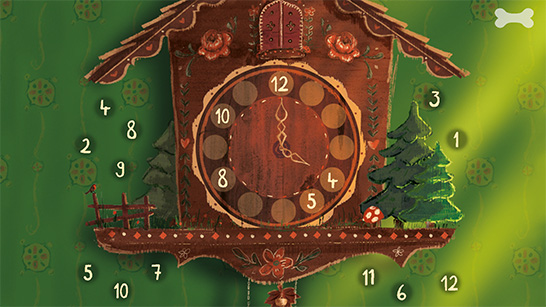### Island

Instruction e.g.: "How many monkeys do you see?
Tap on the correct number!"
Mathematisch Basiskompetenzen: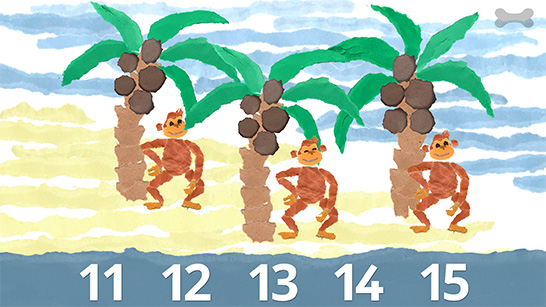### Number Line

Instruction e.g.: "A few of the balls are missing numbers. Push the numbers to the correct place."
Basic Mathematical Skills:
Basic Non-Numerical Skills: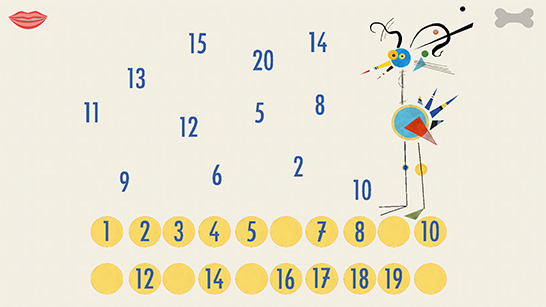### Ducks

Instruction e.g.: "How many ducks are there all together now?
Tap on the correct number!"
Basic Mathematical Skills:### Ghosts

Instruction e.g.: "Catch all the ghosts with red eyes!"
Basic Non-Numerical Skills:### Confetti

Instruction e.g.: "Which object can be seen two times?"
Basic Mathematical Skills:
Basic Non-Numerical Skills:### Chicks

Instruction e.g.: "Tap on the correct result!"
Basic Mathematical Skills: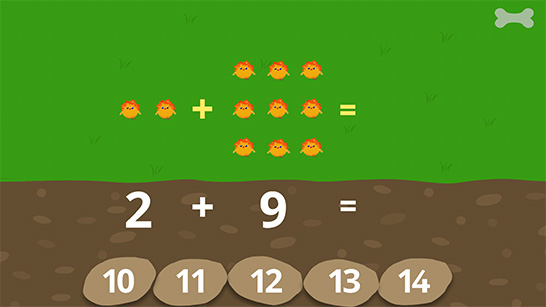### Moles

Instruction e.g.: "Look carefully. Where are the moles?
Do you remember where the moles were? Tap on the correct hills!"
Basic Non-Numerical Skills: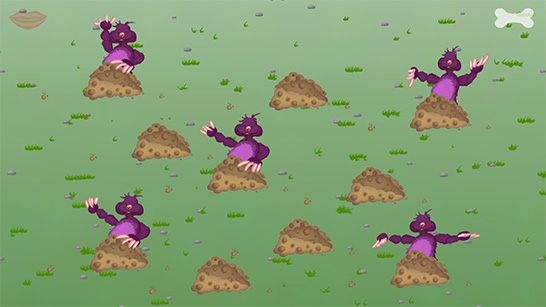### Leprechaun

Instruction e.g.: "Which faces have glasses and a beard?
Tap on the correct faces"
Basic Mathematical Skills: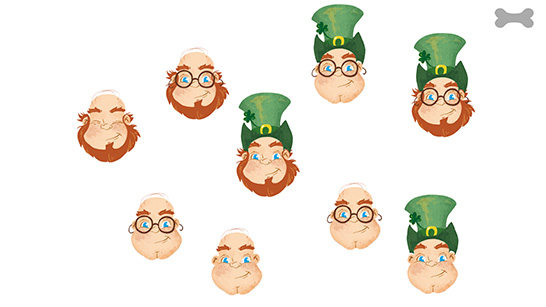### Dragon

Instruction e.g.: "Feed the dragon with the 5!"
Basic Mathematical Skills:
Basic Mathematical Skills: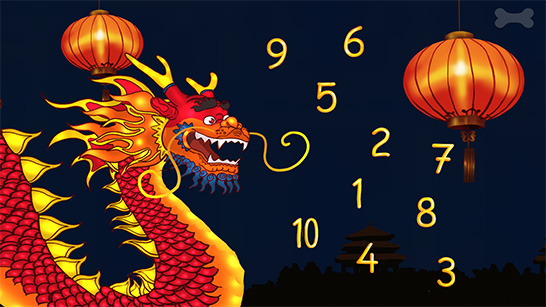### Parrots

Instruction e.g.: "The parrots above are sitting in a certain order. Which parrot is missing in the row above?
Push one of the lower parrots to the correct place!"
Basic Mathematical Skills:
Basic Non-Numerical Skills:### Butterflies

Instruction e.g.: "Which figure only appears once?
Tap on this figure!"
Basic Mathematical Skills:
Basic Non-Numerical Skills: